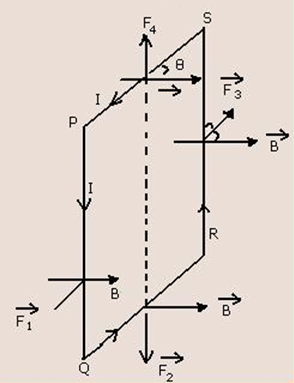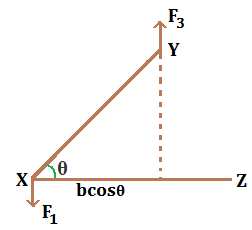# Torque Experienced by a Rectangular Coil in a Uniform Magnetic FieldConsider a rectangular coil, PQRS is carrying current ‘I’ as shown in figure. Let PQ=RS=L and QR=SP=B
Suppose the coil is placed in a uniform magnetic field of strength ‘B’ (magnetic flux density) as shown in figure. Let θ be the angle between the magnetic field and plane of coil.
Now,
Force experienced by PQ, F1=BI.PQ.sin 90º

∴ F= BI.l [perpendicularly outward]

Force experienced by QR, F2=BI.QR.sinθ

∴ F= BIb.sinθ (downward)

Force experienced by RS, F3=BI.RS.sin 90º

∴ F= BI.l [perpendicularly inward]

Force experienced by SP, F_4=BI.SP.sin(π-θ)

∴ F=BI.b.sinθ (upward)

Here, F1 and F3 are equal, opposite and acting at a certain separation,thus they form couple. F2 and F4 are equal, opposite and acting in a straight line. Their effect will be cancelled by the rigidity of the coil.

Torque produced by the coupleIn figure,

τ = F1 or F3 × XZ
τ =BI.lb.cosθ
τ =BI.A.cosθ

If there are ‘N’ turns of coil,
τ =BI.A.cosθ.N
∴ τ =BINAcosθ

This is the required relation.

Do you like this article ? If yes then like otherwise dislike : 19

#### One Response to “Torque Experienced by a Rectangular Coil in a Uniform Magnetic Field”

1.MOHAN GOWDA.S

this one is really help full